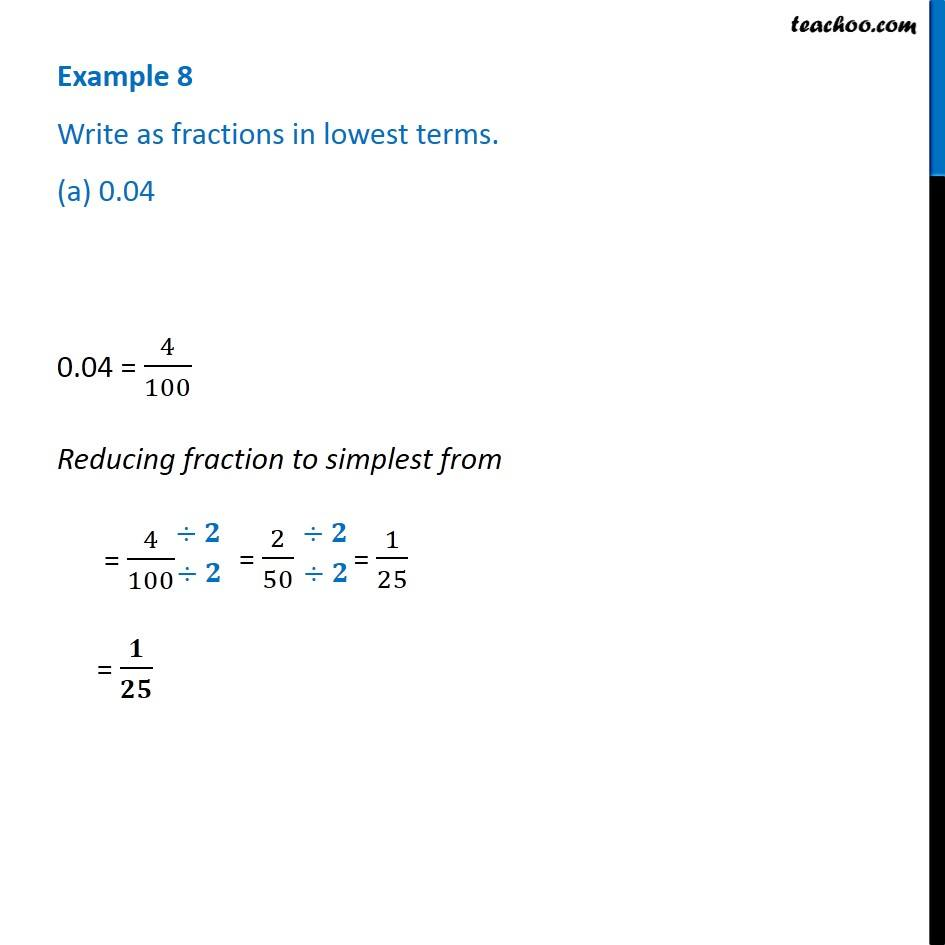1. Chapter 8 Class 6 Decimals
2. Concept wise
3. Decimals to fractions

Transcript

Example 8 Write as fractions in lowest terms. (a) 0.04 0.04 = 4/100 Reducing fraction to simplest from = 4/100 = 𝟏/𝟐𝟓